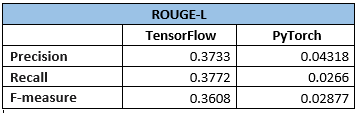# How to debug a Seq2Seq model?

Hi Everyone,

I have a question on debugging a Sequence2Sequence neural network in Pytorch. Here is the scenario:
We have 2 models, one is implemented in Tensorflow and the other in Pytorch. After running 1 epoch for each, we got training loss of 1.32 (Tensorflow) and 2.1 (Pytorch). The problem here is the predicted summaries for Tensorflow code are much closer to the gold summaries than Pytorch predicted summaries. Also, most of the PyTorch model predictions are PAD tokens.
Can anyone help us in debugging the PyTorch seq2seq model to get comparable predictions to Tensorflow predictions?
P.S. -> The TensorFlow code was implemented using Tensorflow 1.0 and we are now trying to replicate it in the newest version of Pytorch.Well, one basic indicator is whether you can overfit your network on a (very) small training dataset. If you can’t get it to overfit, i.e., get a training accuracy of 100% or close to, then your network is not training. Most likely because of some issues in your code.

Do you have a Github link or something to have a look at your code. If it’s not to much, maybe you can post your code here. The important parts are usually the model (with the forward method) and the training loop.